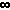Dave's Math Tables: Interest and Exponential Growth(Math | General | Interest and Exponential Growth)

### The Compound Interest Equation

P = C (1 + r/n) nt
where
P = principal (current worth)
C = initial deposit
r = interest rate (expressed as a fraction: eg. 0.06)
n = # of times per year interest in compounded
t = number of years invested

### Simplified Compound Interest Equation

When interest is only compounded once per yer (n=1), the equation simplifies to:
P = C (1 + r) t

### Interest Compounded Continually

When interest is compounded continually (i.e. n -->), the compound interest equation takes the form:
P = C e rt

### Demonstration of Various Compounding

The following table shows the final principal (P), after t = 1 year, of an account initally with C = \$10000, at 6% interest rate, with the given compounding (n). As is shown, the method of compounding has little effect.
 n P 1 (yearly) \$ 10600.00 2 (semi-anually) \$ 10609.00 4 (quarterly) \$ 10613.64 12 (monthly) \$ 10616.78 52 (weekly) \$ 10618.00 365 (daily) \$ 10618.31 continuously \$ 10618.37